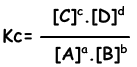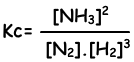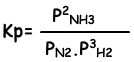EN | ES | DE | NL | RU

## Chemical Equilibrium Cheat Sheet

Chemical Equilibrium Cheat Sheet

Until now, we have learned that reactions take place in one direction. But now we deal with reversible reactions. Reaction, given below, is in closed container;

A(g) + B(g) → C(g) + D(g)

At the beginning, A reacts with B and  produce C and D gas. This is forward reaction and rate of this reaction is written as;

Reaction Rate= kf.[A].[B]

Since concentrations of A and B decrease with time, forward reaction rate also decreases. Moreover, produced C and D molecules collide to each other and form A and B molecules. This is called reverse reaction and rate of it is written as;

Reaction Rate= kr.[C].[D]

Equilibrium constant of following reaction is written as;

aA + bB ↔ cC + dDHomogeneous and Heterogeneous Equilibrium

If matters in equilibrium reaction are all in one phase then it is homogeneous equilibrium, if at least one of the matter has different phase then it is heterogeneous equilibrium. When we write equilibrium equation of heterogeneous equilibrium,we do not write pure solid and pure liquid matters in equilibrium equation.

CaCO3(s) ↔ CaO(s) + CO2(g)

This reaction is heterogeneous equilibrium reaction and we do not write CaCO3(s) and CaO(s) in equilibrium equation.

Equilibrium Constant in terms of Partial Pressure

Concentrations of gases are directly proportional to partial pressure of them. Thus, we can write equilibrium constant in terms of partial pressures.

N2(g) + 3H2(g) ↔ 2NH3(g)

Equilibrium constant of this reaction in terms of concentrations is;Equilibrium constant of this reaction in terms of partial pressure is;1. Affect of Concentration on Equilibrium:

Adding or removing matters into reaction effect equilibrium. For example, adding reactants or removing products increase the yield of product. On the contrary, adding products or removing reactants increase yield of reactants. I other words, in first situation equilibrium shifts to the right and in second situation equilibrium shifts to the left.

2. Affect of Temperature on Equilibrium:

• In an endothermic reaction; increasing temperature shift equilibrium to the right and equilibrium constant increases.
• In an endothermic reaction; increasing temperature shift equilibrium to the left and equilibrium constant decreases.

3. Affect of Pressure on Equilibrium:

• If one of the matters in container  under constant temperature and pressure is removed or added, pressure of the system changes. However, change in the concentration is taken into consideration not pressure.
• Temperature can be changed under constant volume. In this situation even if pressure changes, we consider changes in the temperature while finding equilibrium constant.
• In gas reactions, if there is no change in number of moles, then pressure do not effect equilibrium.

Chemical Equilibrium Exams and Problem Solutions

The Original Author: